blog

# What is the Sharpe Ratio?

#### Options Trading 101 - The Ultimate Beginners Guide To Options

As Seen On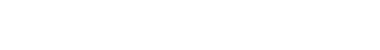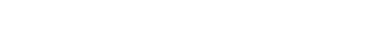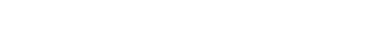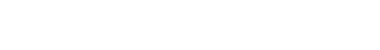by Gavin in Blog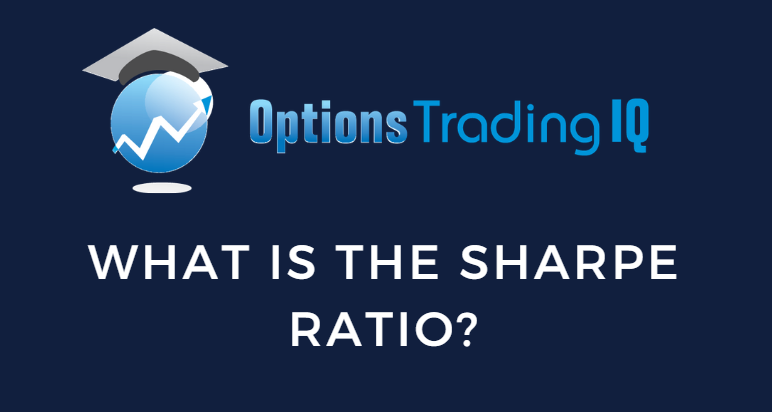## Introduction to the Sharpe Ratio

The Sharpe Ratio is an often-used metric to evaluate a strategy’s performance.

It was conceived by economist William F. Sharpe in 1966 – hence the name.

William Sharpe and Harry Markowitz later won the 1990 Nobel Prize for Economics for their work on evaluating stock-market risk and reward and on valuing corporate stocks and bonds.

Intuitively, you can think of the Sharpe ratio as the risk-adjusted performance of a strategy considering the volatility of the ups and downs in the profit and loss (P&L).

Here we use the word strategy to mean a series of wins and losses from trading a particular method.

While one strategy may sound great with a 20% annual return, what was the drawdown the investor suffered to get this return?

How large were the swings in the equity curve?

It doesn’t sound so great if it had lost 35% at some point before it recovered and gained the 20% return.

Another strategy may also have a 20% annual return but with a minimal drawdown of 5% and a smooth P&L graph.

Both strategies can report a 20% profit potential.

The investor could not tell the difference between the two based on that number.

The Sharpe ratio provides additional information to tell the investor how smooth it was to get that return.

In our example, the second strategy will have a higher Sharpe ratio than the first.

A higher Sharpe ratio denotes better performance when adjusting for risk, as it signifies higher returns relative to the level of risk.

Conversely, a lower Sharpe ratio suggests a lower risk-adjusted performance.

To take another example, suppose an investor was given a choice:

1. Strategy with a 25% annual return with a Sharpe ratio of 0.75
2. Strategy with a 15% annual return with a Sharpe ratio of 1.25

## Which One Would You Choose?

Some investors may choose choice A because, in the end, it results in larger gains.

Other investors may choose choice B because they prefer to make less money in order to avoid the stress of taking on greater risk and potentially watch their account draw down by large amounts.

A Sharpe ratio of 1 to 2 is considered good.

A Sharpe ratio of 3 is considered excellent.

If you see a negative Sharpe ratio, run away as fast as you can.

## Definition Of Sharpe Ratio

The Sharpe ratio is defined as the portfolio’s return in excess of the risk-free rate.

And then dividing that by the standard deviations of those returns.

If the Sharpe ratio is negative, that means that the strategy’s return is less than that of the risk-free rate.

Or the strategy may be losing money with negative returns.

Putting money in US Treasury bills (which is considered a risk-free rate of return) would be more profitable than putting money in a strategy with a negative Sharpe ratio.

The denominator of the Sharpe ratio is the standard deviation of the returns.

The larger the standard deviation, the lower the Sharpe ratio will be.

The smaller the standard deviation, the less variance in the returns, which means a higher and better Sharpe ratio.

You can think of the Sharpe ratio as indicating how consistent the returns are.

The returns are more consistent if there is less variance in the returns, which will increase the Sharpe ratio.

## Example Calculation Of The Sharpe Ratio

Suppose a strategy had the following annual returns in the last five years:

15%
16%
20%
18%
3%

Average annual return: 14.4%

Standard deviation of the annual returns: 6.7%

Average annual risk-free rate: 4%

Annual Sharpe ratio: (14.4 – 4) / 6.7 = 1.6

## Calculating Sharpe Ratio Based On Monthly Data

Suppose a strategy had the following returns in the last five months:

5.70%
5.30%
0.90%
-0.10%
2.50%

Average monthly return: 2.86%

Average annual risk-free rate: 5%

Monthly risk-free rate of return: 5% / 12 = 0.42%

Average monthly return in excess of risk-free rate: 2.44%

Standard deviation of the monthly returns: 6.7%

Monthly Sharpe ratio: 2.44 / 6.7 = 0.36

Annualized Sharpe ratio: 0.36 * sqrt(12) = 1.26

Suppose you are using monthly data to calculate the Sharpe ratio.

In that case, you can get the standard annualized Sharpe ratio by multiplying the monthly Sharpe ratio by the square root of 12, with 12 being the number of months in the year.

## Limitations Of The Sharpe Ratio

The result of the Sharpe ratio calculation can differ depending on whether you are using monthly or yearly data and the length of the timeframe of the data.

Using the standard deviation in its denominator assumes a bell-shaped distribution of returns where it is equally possible to get a return below the average as it is to get a return above the average.

This assumption is not necessarily accurate for certain strategies.

For example, a strategy of picking up pennies in front of a steam roller would not fit that assumption.

The strategy may make very consistent returns for a long time, boosting the Sharpe ratio.

But the Sharpe ratio does not account for those rare outsized losses.

The Sortino and Treynor ratios try to improve this using different divisors.

### How is the Sortino ratio different from the Sharpe ratio?

The Sortino ratio is similar to the Sharpe ratio except that the denominator of the Sortino ratio looks only at the negative returns in order to focus more on downside variance.

### How is the Treynor ratio different from the Sharpe ratio?

The Treynor ratio’s denominator is the asset’s beta instead of the standard deviation.

Beta is a measure of systemic risk and describes how the volatility correlates to that of the market as a whole.

## Conclusion

When evaluating a particular strategy, looking at the rate of return or its win rate is insufficient.

It is also important to look at how tradable the strategy is.

The higher the Sharpe ratio, the more comfortable it is to trade because one is getting the returns without large volatility or risk as compared to another similar strategy with a lower Sharpe ratio.

If a strategy publishes some performance data, you can now calculate its Sharpe ratio on your own and evaluate if this is a strategy that fits your risk tolerance.